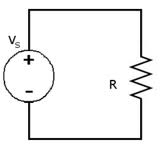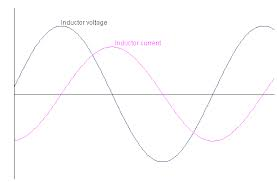## Resistive - Inductive and Capacitive Circuits Assignment Help

Assignment Help: >> AC Circuits - Resistive - Inductive and Capacitive Circuits

Resistive, Inductive and Capacitive Circuits:

AC through Pure Resistive Circuit

Applied voltage,    v = Vm  sin ωt

The resultant current, i

i = V/ R            =     (V m  / R )sin ωt = Im sin ωt

Current, i is in phase with applied voltage, v. The waveforms and phasor diagram are illustrated in Figures (a)and (b), respectively.Figure: Resistive CircuitFigure: Current and Voltage Waveforms

AC through Pure Inductive Circuit

The circuit is illustrated in Figure. Again the applied voltage is given by

v = Vm  sin ωt

v = L  (di /dt)

Now,

v = Vm  sin ωt

∴          Vm sin ωt = L ( dt/ di)

∴          di = (Vm / L ) sin ωt dt

Integrating both sides, we get

i = Vm / L ∫sin ωt dt

= Vm/ ωL (- cos ωt) = - (Vm/ ωL) cos ωt

=  (Vm /ωL) sin (ωt - (π/2))

=  (Vm/ X L) sin ( ωt -( π /2))

where XL = inductive reactance = ωL in ohm, if we put    Vm  / X L = Im , then

i = I msin ( ωt - (π /2))

 AC through Pure Capacitor Parallel RLC Circuit Series RLC Circuit